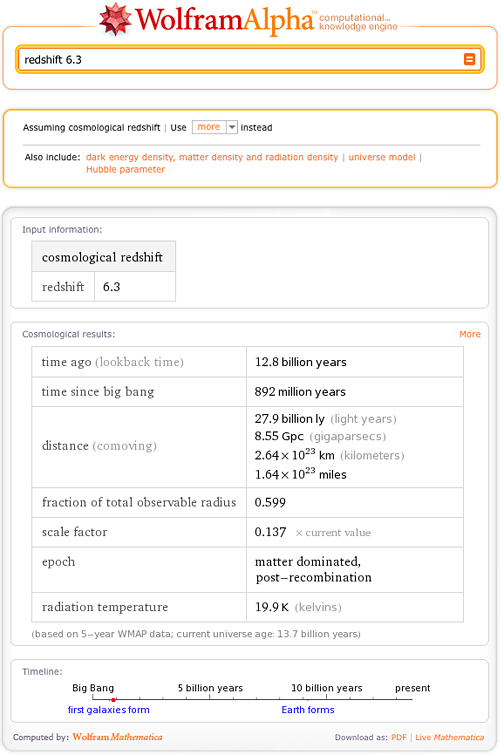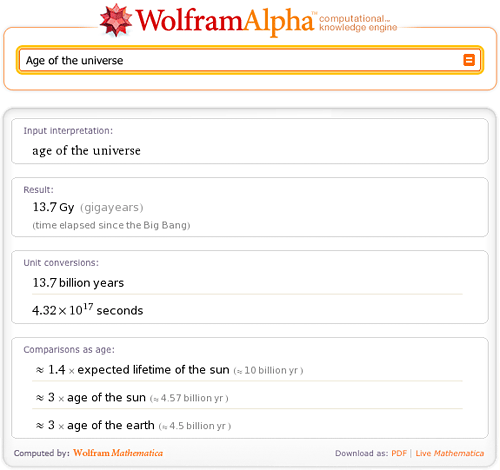# Cosmology with Wolfram|Alpha

December 18, 2009 —Comments Off

When astronomers observe a distant object in the universe, how do they know how far away it is? One method involves the object’s redshift.

What is redshift? It is a shift in the wavelength of electromagnetic radiation toward the longer-wavelength (red) end of the spectrum. Astronomers measure redshift by looking at the spectrum of light from a given distant object.

Type “redshift 6.3” into Wolfram|Alpha.The assumption pod at the top indicates that Wolfram|Alpha has interpreted our “redshift” query as “cosmological redshift”. The “more” menu there lets you access alternate interpretations.

Cosmological redshift is the stretching of light waves due to the expansion of space. Assuming standard measured values for various cosmological parameters describing the expansion of space, Wolfram|Alpha can use the redshift to compute how long ago the light was emitted. In the “Cosmological results” pod, we see that the light was emitted 12.8 billion years ago. Our universe is about 13.7 billion years old:So this light must have been emitted when the universe was very young. The timeline graphic at the bottom gives us an idea about how far back we are looking.

How far away from us is the object that emitted this light? An object whose cosmological redshift is 6.3 is 27.9 billion light years away from us—assuming it still exists. This is even though the light from the object has only traveled 12.8 billion light years—and the universe is only 13.7 billion years old! The universe is expanding!

To be able to see an object in the universe we need light from that object to reach us. So if the universe has been around for only 13.7 billion years we can only see those parts of the universe from which light could have reached us in that time. The distance to the edge of the observable universe, called the horizon, is about 46.5 billion light years. In Wolfram|Alpha’s redshift results, the “fraction of total observable universe” figure is the ratio of the comoving distance to the horizon.

The “scale factor” result is a measure of the size of the universe. The value of the scale factor is usually taken to be equal to 0 at the time of the Big Bang and 1 at the current time. So a value of 0.137 tells us that 12.8 billion years ago the universe was a little more than a tenth of its current size.

We’ve only touched on a few of Wolfram|Alpha’s abilities that astronomers and cosmologists can use. You can try looking at different redshift values. You can also try inputs like “5 billion years after the Big Bang“.

Wow, that is so cool! I used Wolfram to do some simple standard deviation evaluations for our Infinite Money Machine and it works great! Thank you!

Can’t wait for the next development!

Posted by George December 18, 2009 at 3:48 pm

I wonder if this measurement is exact or just estimate. Statistically speaking, what is the margin of error for this measurement?

Posted by bob December 28, 2009 at 1:53 am

Fantastic! What does “6.3” refer to? Does it mean that the observed wavelength is 6.3 percent longer, or something like that?

Posted by Tim December 29, 2009 at 9:37 pm

After looking at several programs on the TV after the Nobel prize ceremony I asked myselt: How are they certain that the redshift is caused by speed only. During theese enormous distances perhaps the lightwaves get a bit tired and as a result lengthen themselves. ?

Posted by P O Nordström February 27, 2012 at 11:09 am